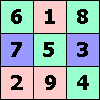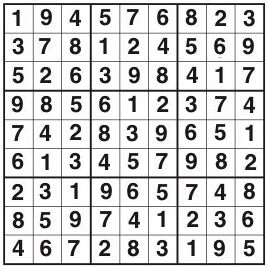ONE TO NINE by Andrew Hodges### Solution to the ultimate Sudoku

#### This last puzzle does not appear in the original British Short Books 2007 edition, but is included in the 2008 American and Canadian editions.A: 1 This is an even power of 9, and every even power of 9 ends in 1. B: 4. First, count the number of choices for the 4 numbers which remain in position. There are 9!/(4! × 5!) = 126 choices. For each such choice, the other 5 numbers are deranged. This can be done in 5! × (1/2! &minus 1/3! + 1/4! &minus 1/5!) = 44 ways. The total number is 126 × 44 = 5544. C: 7. Note that modulo 100, the powers of 2, with the exception of 20 and 21, run round a cycle of length 20. As a trillion is a multiple of 20, the last two digits of the trillionth power of 2 are the same as the same as those of the 20th power, namely 76. (Equivalently, use the fact that all powers of 76 end in 76.) D: 8. If the letters were all distinct there would be 9! anagrams. As there are 3 N's, 2 E's and 2 O's, this is an overcounting by a factor of 3! × 2! × 2! = 24. The total is 9!/24 = 15120. Then 5 + 1 + 2 = 8. E: 1 1/666 = 15/9990 = 0.0015015015015... repeating in a cycle of 3 after the initial 0. As 666 is a multiple of 3, the 666th digit is the same as the 3rd digit, i.e. 1. F: 2. The number is just 6! = 720. G: 5. Consider first the six men. The number of pairings in given by 6!, divided by three factors of 2 to account for the fact that the pair (AB) is the same as (BA), and then by 3! to account for the fact that the pairing (AB)(CD)(EF) is the same as (AB)(EF)(CD) etc. This gives 15. The women likewise, giving a total of 15 × 15 = 225 pairings. H: 9. Similarly to G, the number is 12! divided by (26 × 6!), giving 10395. I: 2. As in B, but now it is 9!/(3! × 6!) = 84 for the selection of 3 items, multiplied by the number of derangements of 6, which is 6! × (1/2! &minus 1/3! + 1/4! &minus 1/5! + 1/6!) = 265. The total is 22260. J: 4. The number is 7! divided by 6 to account for the 3 A's, which gives 840. K: 7. In year 1 B.C.E. the earth was (on this theory) 4003 years old. In the following year, 1 C.E., it was 4004. So 1996 years later, in 1997, it was 6000 years old. L: 3. In binary notation, the Mersenne prime consists of a string of 32582657 1's. Converting into octal notation, each triplet 111 becomes a 7, except for the 2 odd 1's at the beginning which become a 3. (32582657 = 2 modulo 3) M: 7. Look at the Fibonacci numbers modulo 100, i.e. keeping only the last two digits. They run in a cycle of length 300. As a trillion is 300 × 3333333333 + 100, the last two digits of the trillionth Fibonacci number are the same as those of the 100th Fibonacci number, which are 75. N: 2. The first Fibonacci number is 1 and the noughth is 0. Working backwards, using the defining relationship, the minus-first must be 1, the minus-second must be −1, and the minus-third is 2. O: 8. Use the cycle noted in the solution to C. The shows that 32582657th power of 2 ends in the same two digits as the 17th power of 2, which are 72. The Mersenne prime ends in 71. P: 6. Any number of the form 24n ends in a 6. Q: 5. The numbers are 14, 23, 34, 42, 50 and the the next stop on the New York City subway is at 59th Street. R: 1. This first needs Euclid's algorithm to find the HCF of 17 and 32040: 32040 = 1884 × 17 + 12; 17 = 12 + 5; 12 = 2 × 5 + 2; 5 = 2 × 2 + 1. Now work backwards: 1 = 5 − 2 × 2 = 5 − 2 × (12 - 2 × 5) = 5 × 5 − 12 × 2 = 5 × (17 − 12) − 12 x 2 = 5 × 17 − 7 × 12 = 5 × 17 − 7 × (32040 − 1884 × 17) = (5 + 7 × 1884) × 17 modulo 32040 = 13193 × 17 modulo 32040. So D =13193. S: 5. We need C = 6011 modulo 143. This could be done directly, but there is a way of breaking it down which minimises arithmetic with large numbers: 602 = 3600 = 25 × 143 + 25, so 602 = 25 mod 143. Likewise 604 = 252 = 625 = 4 × 143 + 53 = 53 modulo 143. Squaring again, 608 = 532 = 2809 = 19 × 143 + 92 = 92 modulo 143. Now 603 = 60 × 25 = 1500 = 10 × 143 + 70; 6011 = 603 × 608 = 70 × 92 = 6440 = 45 × 143 + 5 = 5 modulo 143. T: 3. Apply Euclid's algorithm. 12345679 = 888888 × 13 + 790135. 888888 = 790135 + 98753. 790135 = 8 × 98753 + 111. 98753 = 889 × 111 + 74. The HCF of 111 and 74 is 37. Or, use prime factors 888888 = 2 × 2 × 2 × 3 × 7 × 11 × 13 × 37, 12345679 = 37 × 333667. U: 6. This is easier to spot when Y has been solved. The numbers 19, 104, 22, 227, 129, 193, 241 are 191, 192, 194, 198, 1916, 1932, 1964 modulo 257, i.e. each is the square of the last, modulo 257. The next one is 256. V: 8. 1/12345679 = 81/999999999 = .000000081 recurring in cycles of 9. 65537 = 8 (modulo 9) so the 65537th digit is the same as the 8th digit, i.e. 8. W: 9. This is an odd power of 9, and every odd power of 9 ends in 9. X: 4. The Gregorian calendar repeats in periods of 400 years, which make an exact number of weeks. So January 802701 is just like January 1901. 1900 was not a leap year, so 1 January 1901 was a Tuesday, and the first Friday fell on 4 January. Y: 7. This is like the Spectrum sequence of chapter 7, but using modulus 257. The numbers 19, 104, 177, 22, 161, 232, 39 are 191, 192, 193, 194, 195, 196, 197 modulo 257. The next is 227. Z: 3. The Fibonacci number at the start of Nineteen Eighty-Four is 13: 'the clocks were striking thirteen'.